Courses

# Laplace Transform - Practice GATE Notes | EduRev

## : Laplace Transform - Practice GATE Notes | EduRev

Introduction

• Till now, we have been dealing with continuous and discrete domains. Then we studied the relationships involved using the transform domains. A system actually operates in a natural domain but it can be well understood in transform domains.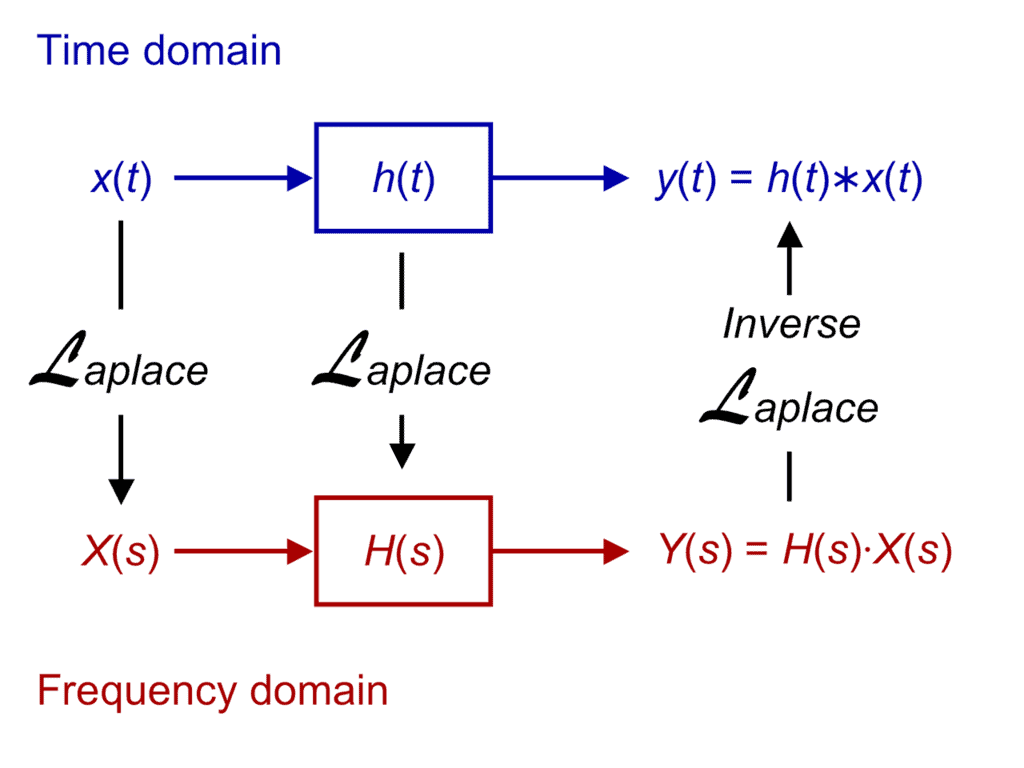Laplace Transform

• The advantage of transform domains is that a few of the properties which may not be observed in natural domains are clear in transform domains. Most of the LTI-Systems act in the time domain but they are more clearly described in the frequency domain instead.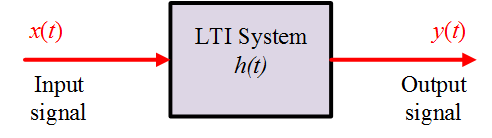LTI System

• Till now, we have seen the importance of Fourier analysis in solving many problems involving signals and LTI systems. Now, we shall deal with signals and systems which do not have a Fourier transform.
• But what was so special about Fourier transform in case of LSI systems?
We found that the continuous-time Fourier transform (F.T.) is a tool to represent signals as linear combinations of complex exponentials. The exponentials are of the form est with s=jω and ejw is an eigenfunction of the LSI system. Also, we note that the Fourier Transform only exists for signals which can absolutely integrated and have finite energy.
• This observation leads to a generalization of continuous-time Fourier transform by considering a broader class of signals using the powerful tool of "Laplace transform".
• It will be trivial to note that the L.T. can be used to get the discrete-time representation using relevant substitutions. This leads to a link with the Z-Transform and is very handy for a digital filter realization/designing. Also, it will be helpful to note that the properties of Laplace Transform and Z-Transform are quite similar.

Laplace Transform

➢ Definition

• The response of a Linear Time-Invariant system with impulse response h(t) to a complex exponential input of the form est can be represented in the following way: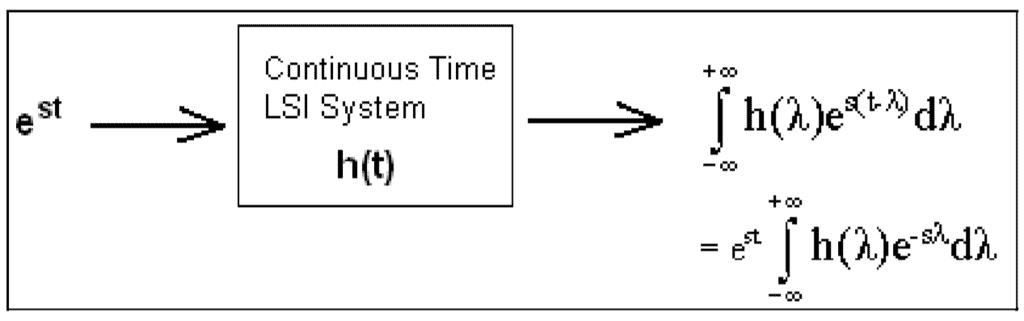•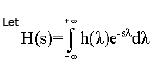Where H(s) is known as the Laplace Transform of h(t).
• We notice that the limits are from [ -∞ to + ∞] and hence this transform is also referred to as Bilateral or Double-sided Laplace Transform. There exists a one-to-one correspondence between the h(t) and H(s), i.e. the original domain and the transformed domain. Therefore L.T. is a unique transformation and the 'Inverse Laplace Transform' also exists.
• Note that est is also an eigenfunction of the LSI system only if H(s) converges. The range of values for which the expression described above is finite is called the Region of Convergence (ROC). In this case, the Region Of Convergence is Re(s) > 0.
• Thus, the Laplace transform has two parts which are, the expression and region of convergence, respectively. The region of convergence of the Laplace transform is essentially determined by Re(s). Here onwards, we will consider trivial examples for a better understanding of the ROC.

➢ Example of Laplace Transform

• Consider that the impulse response h(t) = etu(t).
Thus we notice that by multiplying by the term u(t) we are effectively considering the unilateral Laplace Transform whereby the limits tend from 0 to +∞.
• Also, we notice that h(t) is not Fourier transformable as it is not absolutely integrable.
Consider the Laplace transform of h(t), as shown below: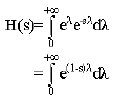• As stated earlier, the symbol s is a complex number and is defined as s = σ + jΩ..
Substituting s in the above equation we get: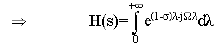• Observing the above equation closely, we realize that firstly H(s) converges if and only if,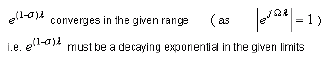• We analyze the part (1-σ) as follows:
For a decaying e(1-σ)λ it is essential that (1 - σ ) < 0. This implies that ( σ > 1) which means that the Real part of 's' is greater than '1' which is also denoted as Re(s) > 1.
• This is what defines the " Region of Convergence " in an S-Complex Plane. The ROC of the Laplace Transform is always determined by the Re(s). The ROC, in general, gives us an idea of the stability of a system and is also a representation of the poles-zero plot of a system. It is essential to note that the ROC never includes poles.
• Evaluation of the integral yields: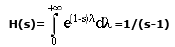We observe that there is a single pole at s=1. Since the Region of Convergence cannot contain poles; therefore ROC starts from '1' and tends outwards to infinity. est in physical systems: We consider the real part of est, where,
s = σ + jΩ.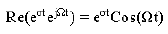• Such a response is visible in RLC (Resistance-Inductance and Capacitance) systems. It is not only visible in the electrical field but also in other disciplines like the mechanical field. In such cases, the above expression is multiplied by a polynomial or a combination of such expressions.
• What is the need to consider the region of convergence while determining the Laplace transform?
If we consider the signals e-atu(t) and -e-atu(-t) we note that although the signals are differing, their Laplace Transforms are identical which is 1/( s+a). Thus we conclude that to distinguish L.T's uniquely their ROC's must be specified.

A few important properties of the ROC are listed below:

• The ROC of F(S) consists of strips parallel to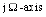in complex variable plane (S-plane)We know that the ROC is dependent only on the real parts of 'S' which is 'σ', therefore the property.
• The ROC does not contain any poles. Since if this happens then the Laplace Transform becomes infinity. For example, if the F(S) = 1/(s-1) then the ROC cannot contain s = 1 because at this point the L.T becomes infinity.
• If h(t) is a time-limited signal and is Laplace Transformable, then its ROC will be the entire S-plane.
Example: the ROC for δ(t) is the entire S-plane.
• The region of convergence is always between two vertical lines in s-plane. These vertical lines need not be in finite region. But note that the ROC is always simply-connected but not multiply-connected in the s-plane.
This fact can be explained by the following illustration: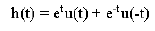Let H1(s) and H2(s) be the respective Laplace transforms of the first and second terms.H1(s) and H2(s) converge in the region Re(s) > 1 and Re(s) < 1 respectively. But, h(t) doesn't have any Laplace transform due to no common ROC where both H1(s) and H2(s) converge.
• Consider another example on ROC
Let us consider another example which illustrates the need to specify ROC for completely defining the Laplace transform of a given function:
h1(t) = etu(t)
h2(t) = -etu(-t)
• We will use the concepts gathered till now to determine the ROC's of the above signals after computing the respective Laplace Transforms.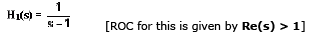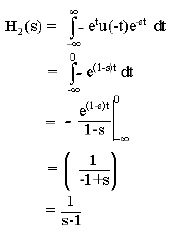• Thus the ROC of H2(s) is given by Re(s) < 1;provided Re(1-s) > 0.
Thus, two different functions may have the same expressions but correspond to different ROC.
ROC's are given as: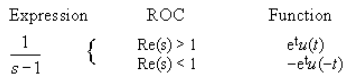Conclusion

In the lecture, you have learnt:

•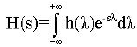is called the Laplace Transform of h(t) and the Region of Convergence (ROC) of the Laplace transform is essentially determined by the real part of the complex number 's' denoted as Re(s).
• Two different functions may have the same Laplace Transform, so the only way to uniquely describe them is by means of ROC.
• The ROC consists of strips parallel to the Jωaxis, it does not include poles and is always simply-connected.
Offer running on EduRev: Apply code STAYHOME200 to get INR 200 off on our premium plan EduRev Infinity!